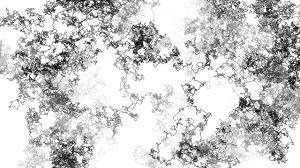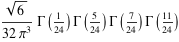# The most surprising numberThe most surprising number I’ve seen in mathematics is deeply connected with the notions of randomness and dimensionality. The first two numbers in the series that lead to this number are: 1, 1. So far so easy, one is a pretty straightforward number. To begin, I’ll reveal where these two ones come from.

Imagine a line. Imagine that line divided into equal segments. I now place a pin in the middle segment. Now I’m going to start moving this pin. Where I move it to depends on the flip of a fair coin; heads I move it left, tails I move it right. That’s the setup. Now the question: what is the probability that the pin will visit a particular segment? The answer is that, given enough coin tosses, whichever segment is selected the pin will visit it with certainty, that is the probability equals one. This probability is the first number in this series of interest. The the next number in the series, also a one.

This second number is derived from the same problem as the first with the difference that we are no longer dealing with a line, but a plane divided into equal sized square segments. Instead of a coin we randomly pick a direction to move in. Pick a starting point on a flat surface. Pick an end point.  The probability that a pin, moving randomly, will eventually reach the chosen end-segment is one. No matter which end-segment you pick. Another way of putting this is that a pin moving randomly will eventually visit every single segment possible. This result is the same as for the line.

The trend in the series is becoming apparent, the number in the series at position N is the answer to the question:

What is the probability that a random walk on a lattice in N dimensions will visit an arbitrary point x?

So for one dimension, this probability was one. The same for two dimensions. Any guesses for three?

The answer for three dimensions is: 0.3405373296 . . .

This is the most surprising number I’ve ever seen. It may mostly be surprising to me as I knew and appreciated why for one and two dimensions the value is unity. On thinking about what the number could be for higher dimensions I imagined it would either remain one or decay with some series that involved a relatively simple relationship with π. The expression for this number in three dimensions is not straighforward:In truth I find it difficult to explain just why this seems so odd to me. The most striking feature to me is that it happens in the transition from two to three dimensions, the space in which we naturally find ourselves. Could it be that random collisions in two dimensions does not allow for much fluidity, and collisions in four or more are far too infrequent to allow for many interesting reactions? Just a thought…

For those that are interested the value for four dimensions is: 0.193206 . . .

and this series of numbers is known as Pólya’s Random Walk Constants.